Miscellaneous

Chapter 9 Class 11 Sequences and Series
Serial order wise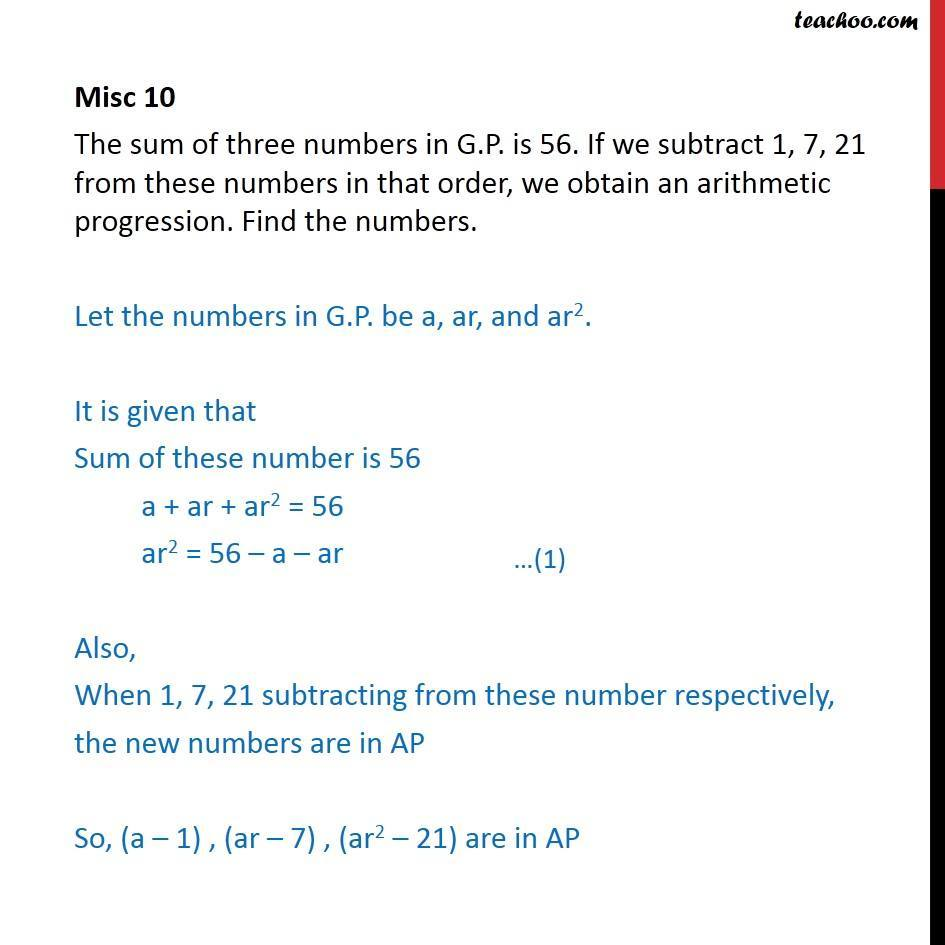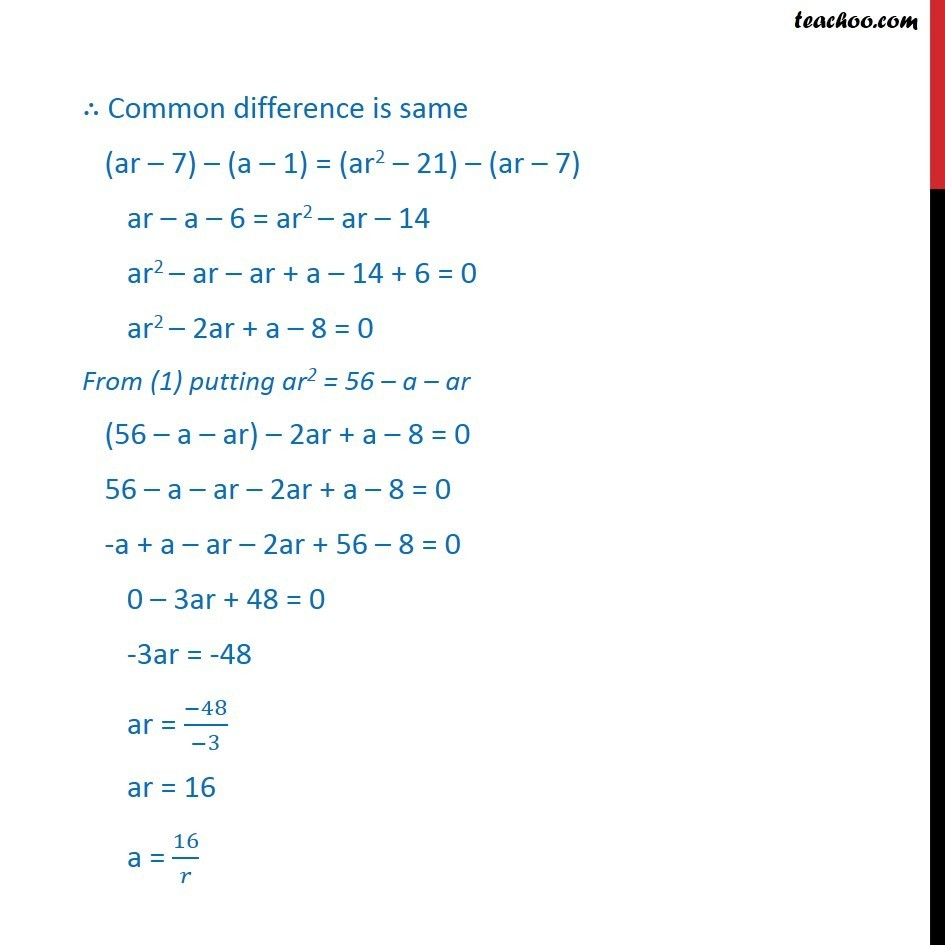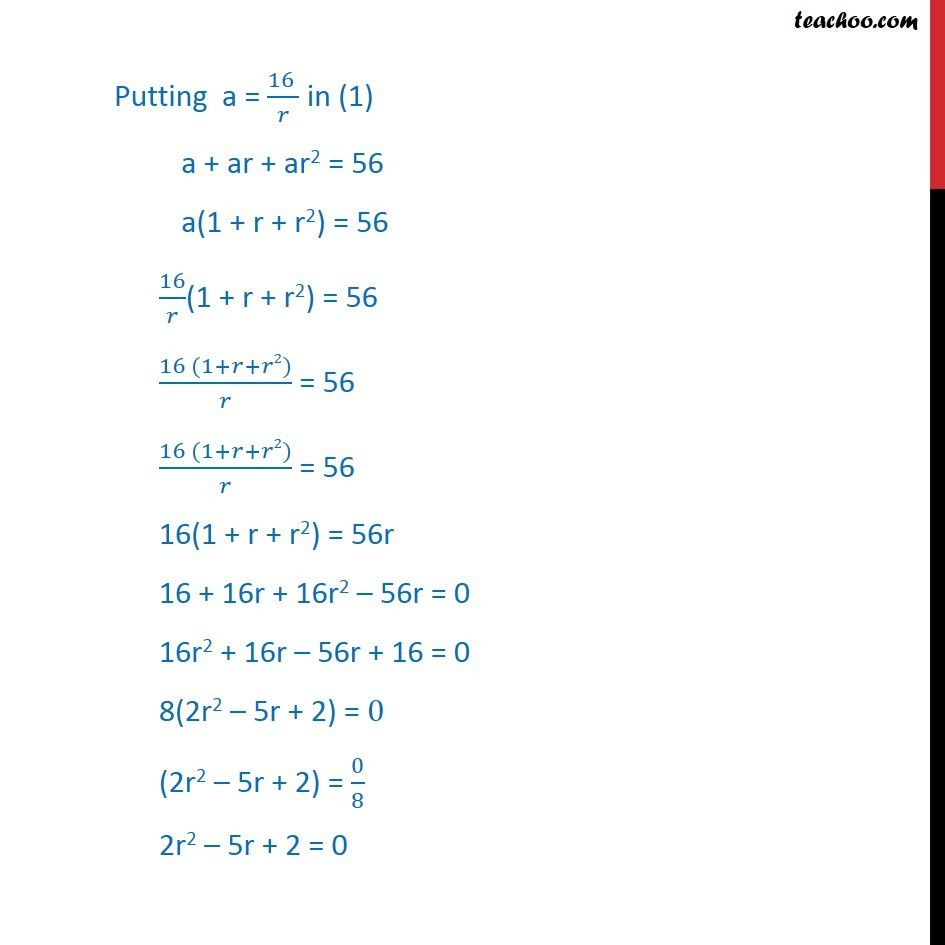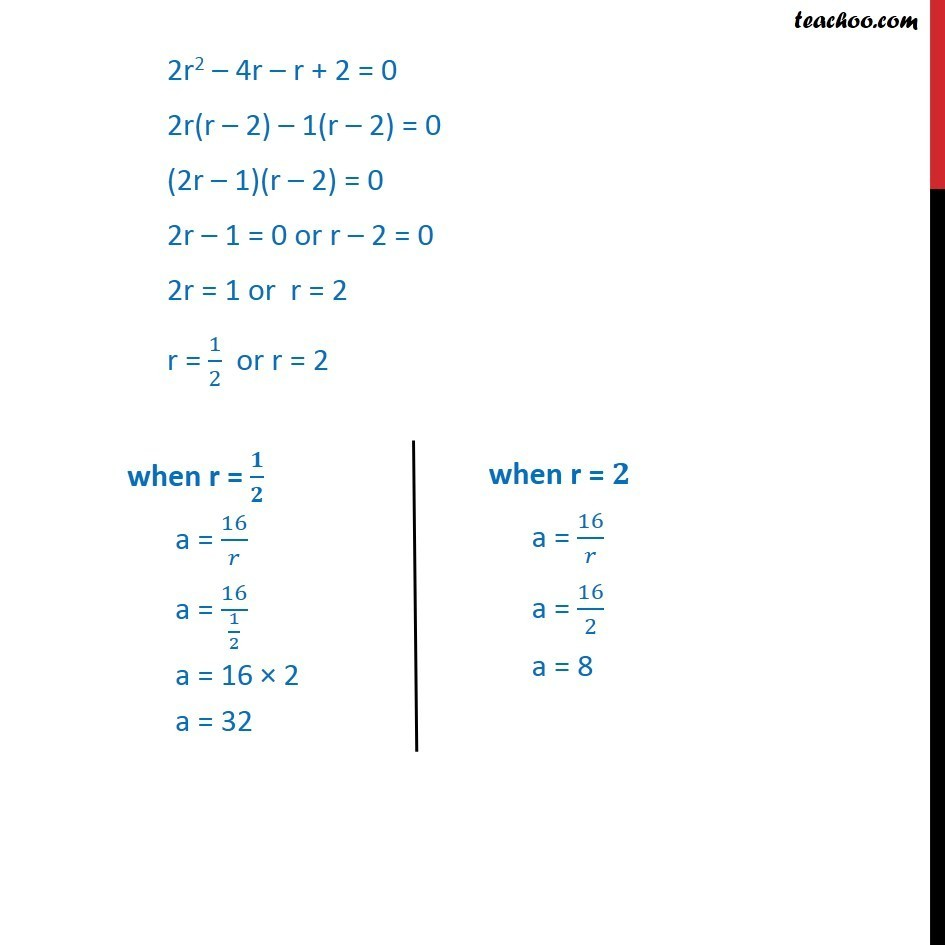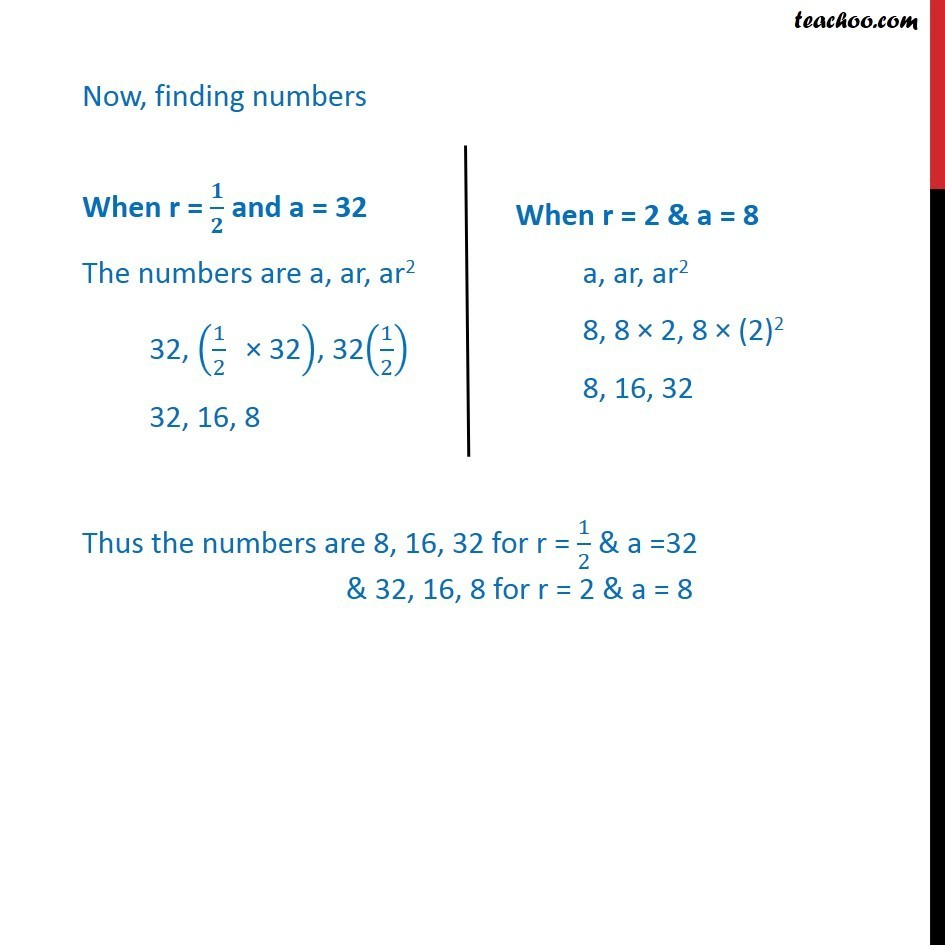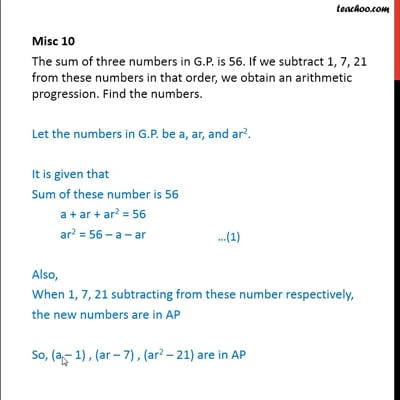This video is only available for Teachoo black users

Maths Crash Course - Live lectures + all videos + Real time Doubt solving!

### Transcript

Misc 10 The sum of three numbers in G.P. is 56. If we subtract 1, 7, 21 from these numbers in that order, we obtain an arithmetic progression. Find the numbers. Let the numbers in G.P. be a, ar, and ar2. It is given that Sum of these number is 56 a + ar + ar2 = 56 ar2 = 56 a ar Also, When 1, 7, 21 subtracting from these number respectively, the new numbers are in AP So, (a 1) , (ar 7) , (ar2 21) are in AP Common difference is same (ar 7) (a 1) = (ar2 21) (ar 7) ar a 6 = ar2 ar 14 ar2 ar ar + a 14 + 6 = 0 ar2 2ar + a 8 = 0 From (1) putting ar2 = 56 a ar (56 a ar) 2ar + a 8 = 0 56 a ar 2ar + a 8 = 0 -a + a ar 2ar + 56 8 = 0 0 3ar + 48 = 0 -3ar = -48 ar = ( 48)/( 3) ar = 16 a = 16/ Putting a = (16 )/ in (1) a + ar + ar2 = 56 a(1 + r + r2) = 56 16/ (1 + r + r2) = 56 (16 (1+ + 2))/ = 56 (16 (1+ + 2))/ = 56 16(1 + r + r2) = 56r 16 + 16r + 16r2 56r = 0 16r2 + 16r 56r + 16 = 0 8(2r2 5r + 2) = 0 (2r2 5r + 2) = 0/8 2r2 5r + 2 = 0 2r2 4r r + 2 = 0 2r(r 2) 1(r 2) = 0 (2r 1)(r 2) = 0 2r 1 = 0 or r 2 = 0 2r = 1 or r = 2 r = 1/2 or r = 2 Now, finding numbers Thus the numbers are 8, 16, 32 for r = 1/2 & a =32 & 32, 16, 8 for r = 2 & a = 8